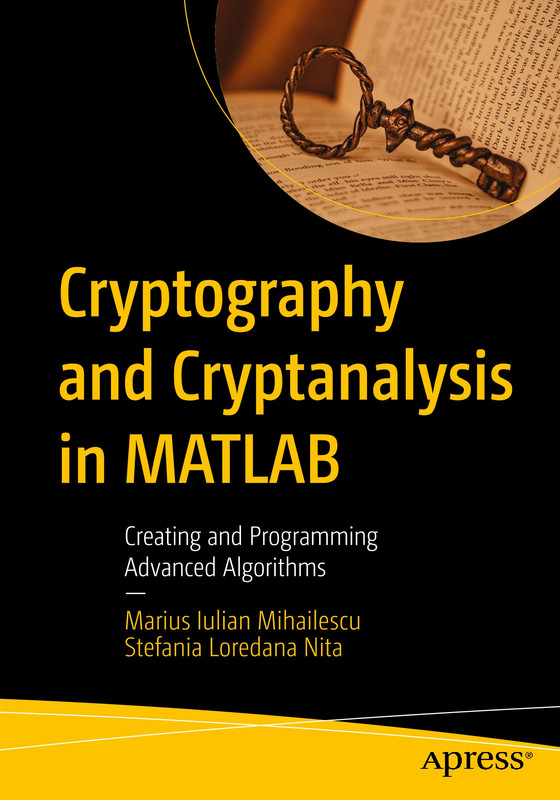# Cryptography and Cryptanalysis in MATLAB Creating and Programming Advanced Algorithms

Discussion in 'Misc.' started by Dl4ever, Sep 12, 2021.

Tags:
1. ### Dl4everActive Member

Joined:
Sep 13, 2020
Messages:
341
55
Trophy Points:
28
Gender:
Malepdf, epub | 2021 | ISBN: ‎ B09G11J571 | 201 pages | English | 11.79 MB

Master the essentials of cryptography and cryptanalysis and learn how to put them to practical use. Each chapter of this book starts with an introduction to the concepts on which cryptographic algorithms are based and how they are used in practice, providing fully working examples for each of the algorithms presented. Implementation sections will guide you through the entire process of writing your own applications and programs using MATLAB.

Cryptography and Cryptanalysis in MATLAB will serve as your definitive go-to cryptography reference, whether you are a student, professional developer, or researcher, showing how a multitude of cryptographic challenges can be overcome using the powerful tools of MATLAB.

What You Will Learn

• Discover MATLAB's cryptography functions
• Work with conversion mechanisms in MATLAB
Implement cryptographic algorithms using arithmetic operations
• Understand the classical, simple cryptosystems that form the basis of modern cryptography
• Develop fully working solutions (encryption/decryption operations)
• Study pseudo-random generators and their real-life implementations
• Utilize hash functions by way of practical examples
• Implement solutions to defend against practical cryptanalysis methods and attacks
• Understand asymmetric and symmetric encryption systems and how to use them
Leverage visual cryptography, steganography, and chaos-based cryptography

Who This Book Is For

Those who are new to cryptography/analysis. Some prior exposure to MATLAB recommended.

Hidden Content:
You must reply before you can see the hidden data contained here.